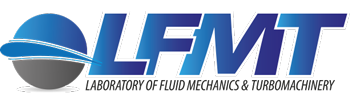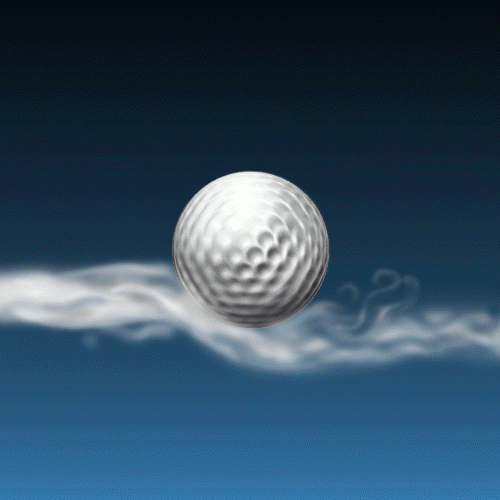1st Cycle of Studies

### (118) Fluid Mechanics

Introduction to fluids and their general properties. Hydrostatic pressure, forces due to it. Description of a flow field: equations of continuity, Euler equations, Bernoulli equation, measurement of flow rate, static and total pressure. Conservation of momentum, applications. Similarity, non dimensional numbers, Buckingham’s Π-theory. Navier-Stokes equations, analytical solutions. 2-D, steady, incompressible, irrotational flow: velocity potential, stream function, Laplace equation, elementary flows, linear superposition of elementary flows, applications. The boundary layer: Prandtl’s equation for laminar flow, integral quantities, boundary layer of a flat plate, boundary layer separation. Laminar, transitional and turbulent flow: description of turbulent flow, turbulence quantities, the effect on the flow, introduction to turbulence modelling. Introduction to Computational fluid mechanics. Flow in pipes: Velocity profiles, pressure drop, Moody diagram. Flow and pressure field in pipe components, nozzles, diffusers, curves, valves. External flow, flow around bodies: cylinders, spheres, prisms of various cross sections, aerodynamic shapes. Compressible flow: Speed of sound, Mach number, isentropic flow, Laval nozzle, compressible flow in pipes. Water hammer in pipes, and problem alleviation techniques.

### (127) Mechanical Engineering Laboratory

Experimental set-up. Accuracy, reliability, repeatability. Systematic and stochastic errors. Statistic methods of measurement analysis. Statistical processing of measurement data. Distributions and error analysis. Error propagation. Introduction to measurement systems: Active and passive transducers. Static and dynamic characteristics of measurement instruments. Calibration parameters: linearity, hysteresis, zero drift and sensitivity. Handling, sampling, data transfer and storage. Tension, acceleration, temperature, heat flux, pressure and flow rate measurement techniques. Liquid in glass, bimetallic, pressure thermometers. Thermocouples. Resistance thermometers. Thermistors. Radiation thermometers. Pyrometers. Problems in temperature measurement in flows. Pressure measurement. Manometers. Piezocrystals, electromagnetic, variable resistance, capacitor, natural frequency and strained element instruments for pressure measurement. Flow velocity measurement. Use of Pitot and Pitot static tubes. Fluid volume measurement. Force and torque distortion and dynamic response measurements. Balancing. Measurements of vibrations and evaluation of the results. Permitted values of dynamic loads for various groups of machines and human beings. Application of the above in the fields of thermodynamics, fluid mechanics and aerodynamics, vibration and machine dynamics and machine tools with laboratory exercises and technical reports.

### (128) Energy Transformation Systems

Energy: Primary, secondary, final energy. Usable energy, losses, reactive power. Terminology and definitions.
Heat production and storage systems: Hot water and steam production boilers, heat pump, refrigerating machines.
Internal Combustion Engines: IC engine classification. Layout and terminology. Function maps. Characteristic sizes. Sizing and use of IC engines in conventional and hybrid propulsion systems.
Introduction to Turbomachinery: Typical layouts and types of turbomachines. Turbomachinery maps and characteristics, dimensional analysis. Cooperation between turbomachines and operation of turbomachines in energy networks.Aircraft Design & Performance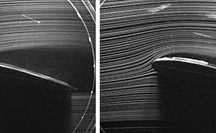Plasma Flow Control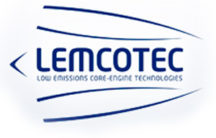LEMCOTECDREAM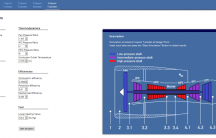Aeroengine Design Application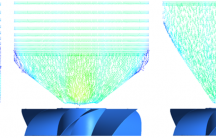Boundary Layer Ingestion Intakes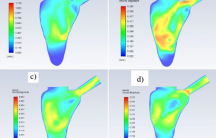Hemodynamics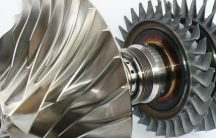Next-MGT